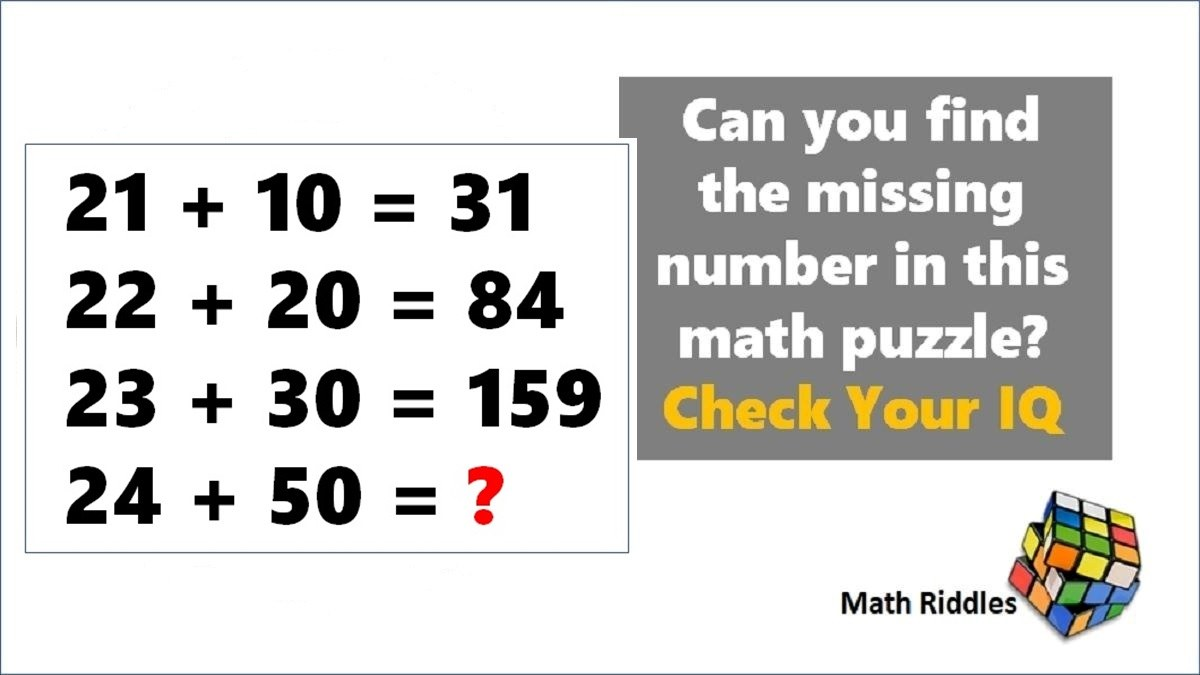# Math Riddles: Only 1% Genius Can Solve These Tricky Mathematics Puzzles in 3 Minutes

Math Riddles Challenge: You are 100% genius if you can find the logic and solve this mathematics puzzle in less than 3 minutes each?Math Riddles: Only 1% Genuis Can Solve This Tricky Mathematics Puzzle in 3 Minutes

Math Riddles: Let us do some brain exercises. Check out these mathematics puzzles that one to be 100% genius to figure out the logic and solve these maths logic puzzles. 99% of people could not solve these mathematical puzzles correctly. We challenge you to solve these!

## Solution

### Maths Logic Puzzle #1

Explanation:

The logic in this mathematics puzzle: Formula -> x + y * 1st Number

=> 5 + 4 = 45, How?

Row 1 => x + y * 1st number = 5 + 4 * 5 = 9 * 5 = 45

Row 2 => x + y * 1st number = 7 + 6 * 7 = 13 * 7 = 91

Row 3 => x + y * 1st number = 9 + 8 * 9 = 17 * 9 = 153

Similarly, we shall find the missing number here.

Row 4 => x + y * 1st number = 8 + 7 * 8 = 15 * 8 = 120

### Maths Logic Puzzle #2

Explanation:

The logic in this mathematics puzzle: Formula -> x + y * Natural Number

=> 21 + 10 = 31, How?

Row 1 => x + y * Natural Number = 21 + 10 * 1 = 31 * 1 = 31

Row 2 => x + y * Natural Number = 22 + 20 * 2 = 42 * 2 = 84

Row 3 => x + y * Natural Number = 23 + 30 * 3 = 53 * 3 = 159

Similarly, we shall find the missing number here.

Row 4 => x + y * Natural Number = 24 + 50 * 4 = 74 * 4 = 296

## Tell us in comments: Did you solve these mathematics puzzles in less than 3 minutes each?

Check out more math puzzles!

Also Read: Math Riddles: Only 1% Can Solve This Math Crossword Puzzle, Difficulty Level Hard

Also Read: Sudoku Solver Tips & Tricks: How to Solve a Sudoku Puzzle Easy, Medium, Hard

Also Read: Math Riddles: Solve This Math Crossword Puzzle, Only with 1 to 9 Numbers

Also Try: Math Riddles: How Many Triangles Do You See In This Picture?

Get the latest General Knowledge and Current Affairs from all over India and world for all competitive exams.
खेलें हर किस्म के रोमांच से भरपूर गेम्स सिर्फ़ जागरण प्ले पर